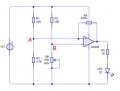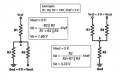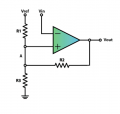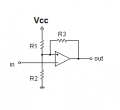# Calculating the hysteresis of a non-inverting Schmitt Trigger using a LM358

#### Ford Prefect

Joined Jun 14, 2010
201
I am wanting to calculate the hysteresis of a non-inverting Schmitt Trigger using a LM358 on the circuit below:According to the resistor/voltage divider, the voltage drop across R1 will be about 8.16 volts and the reference voltage at node A will be about 3.84 volts (12v minus 8.16v)
The voltages at node B will vary between 0 volts and 6 volts.

I have found the links below to calculate the voltage high and voltage low outputs.
1. http://www.pcbheaven.com/drcalculus/index.php?calc=st_nonsym_sp
2. http://hyperphysics.phy-astr.gsu.edu/hbase/Electronic/schmitt.html#c2
3. https://howtomechatronics.com/how-it-works/electrical-engineering/schmitt-trigger

Using the boxes in link 1 and using values of 'Supply voltage' = 5 volts, R1 = 10k, R2 = 10k, R3=10k
I am getting:
V High= 3.333333 Volt
V Low= 1.666667 Volt

and using the same values 'Supply voltage' = 5 volts, R1 = 10k, R2 = 10k, R3=10k in link 2
I am getting:

the trigger voltage will be= 3.3333 Volts
and it will hold until the signal drops to= 0 Volts

When I enter values into these input boxes on these websites I am getting different results or am I missing something!?

So if I use the boxes in link 1 for my circuit ..that is Supply voltage = 12, R1 = 10k, R2 = 4.7k, R3 (RFB) = 220k
I get.. **** = the same, ~~~ = different
V High= 3.953673 Volt ****
V Low= 3.781774 Volt ~~~

whereas when I enter the values in link 2 I get...
V2 = 3.953 volts ****
V'2 = 3.6098 volts ~~~

Also I do not understand what the '||' means in the formulas in link 3
look under Non-Symmetrical Schmitt Trigger
can someone enlighten me?So basically what is the correct formula?

Last edited:

#### Dodgydave

Joined Jun 22, 2012
9,657
Don't forget that the 220K is in parallel with either of R1 or R2, which gives you R1 as 9.5K, and R2 as 4.6K. So your voltage levels will be 3.78V and 3.97V .

To alter the Hysteresis levels make R4 a 1M preset.

•Ford Prefect

#### MrChips

Joined Oct 2, 2009
22,090
Also I do not understand what the '||' means in the formulas
It means "in parallel with".

For example, R1 || R2 is asking for the resistance of two resistors, R1 and R2 connected in parallel.

R1 || R2 = (R1 * R2) / (R1 + R2)

•Ford Prefect

#### Ford Prefect

Joined Jun 14, 2010
201
Don't forget that the 220K is in parallel with either of R1 or R2, which gives you R1 as 9.5K, and R2 as 4.6K. So your voltage levels will be 3.78V and 3.97V .
To alter the Hysteresis levels make R4 a 1M preset
.
Thank you Dodgydave, but how did you calculate the results (3.78V and 3.97V) ?
Can you show me your formula?

It means "in parallel with".
For example, R1 || R2 is asking for the resistance of two resistors, R1 and R2 connected in parallel.
R1 || R2 = (R1 * R2) / (R1 + R2)
Ah, ok, thank you MrChips.I really need to get my head around this!
I see it as this:
Va = R2 || R3 / R1 + R2 || R3 x VRef

and I think expanding this makes this:
Eg. R2 || R3
= (R2 x R3) / (R2 + R3)
= (10k x 10k) / (10k + 10k)
= 100M / 20K = 5000 is the section in RED

R1 + R2 || R3
=
R1 + (R2 x R3) / (R2 + R3)
=
10k + (10k x 10k) / (10k + 10k) = 15000 is the section in GREEN

therefore Va =
5000 / 15000 x 5 (VRef) = 0.33333 x 5 = 1.666666
Is this right, have I missed something?

Last edited:

#### MrChips

Joined Oct 2, 2009
22,090
Without having to do the math, 10k || 10k would be half of 10k = 5k.

#### Jony130

Joined Feb 17, 2009
5,186
There is a error in the equation in link 3:

For this circuit :When opamp output is at Vcc the voltage at point A is

VH = Vcc * R3/( R1||R2 + R3 )

So for your circuit we have:

R1 = 10kΩ; R3 = 4.7kΩ; R2 = 220kΩ and Vcc = 12V

Va = 12V * 4.7kΩ/( 9.56kΩ + 4.7kΩ ) = 3.95V

But when opamp is at low state Vout ≈ 0V the voltage at point A is equal to:

VL = Vcc * (R2||R3)/( R1 + R2||R3 )

VL = 12V *4.6kΩ/(10kΩ + 4.6kΩ ) = 3.78V

#### BobTPH

Joined Jun 5, 2013
2,584
When you put in the correct values, both calculators give you nearly the same answers. But you are not putting in the correct values, because your R1, R2 and R3 are not the same as those in the links.

link1: R1 = 10k, R2 = 4.7K, Rfb = 220K

link2: R1=4.7K, R2 = 10K, R3 = 220K

link3: R1=10K, R2=220K, R3 = 4.7K

Not sure why the answers differ though.

Bob

#### Jony130

Joined Feb 17, 2009
5,186
In link 2 they assume symmetrical supply for a opamp. And this is why v2' is 0V

#### Ford Prefect

Joined Jun 14, 2010
201
There is a error in the equation in link 3:
For this circuit :
View attachment 155720
When opamp output is at Vcc the voltage at point A is
VH = Vcc * R3/( R1||R2 + R3 )
So for your circuit we have:
R1 = 10kΩ; R3 = 4.7kΩ; R2 = 220kΩ and Vcc = 12V
Va = 12V * 4.7kΩ/( 9.56kΩ + 4.7kΩ ) = 3.95V
But when opamp is at low state Vout ≈ 0V the voltage at point A is equal to:
VL = Vcc * (R2||R3)/( R1 + R2||R3 )

VL = 12V *4.6kΩ/(10kΩ + 4.6kΩ ) = 3.78V
don't you mean:
VL = 12V *4.7kΩ/(10kΩ + 4.7kΩ ) = 3.84V ?

#### Jony130

Joined Feb 17, 2009
5,186
don't you mean:
VL = 12V *4.7kΩ/(10kΩ + 4.7kΩ ) = 3.84V ?
No,

VL = Vcc * (R2||R3)/( R1 + R2||R3 )

R2||R3 = 4.7kΩ||220kΩ = (4.7kΩ x 220kΩ)/(4.7kΩ + 220kΩ) = 4.601kΩ

#### Ford Prefect

Joined Jun 14, 2010
201
When you put in the correct values, both calculators give you nearly the same answers. But you are not putting in the correct values, because your R1, R2 and R3 are not the same as those in the links.
link1: R1 = 10k, R2 = 4.7K, Rfb = 220K
link2: R1=4.7K, R2 = 10K, R3 = 220K
link3: R1=10K, R2=220K, R3 = 4.7K
Not sure why the answers differ though.
Bob
Yes, I am aware that the references to the resistors in link 1 and link 2 are 'the other way around' but this was my original question....which formula is correct and what should the results be?

#### Ford Prefect

Joined Jun 14, 2010
201
No,
VL = Vcc * (R2||R3)/( R1 + R2||R3 )
2||R3 = 4.7kΩ||220kΩ = (4.7kΩ x 220kΩ)/(4.7kΩ + 220kΩ) = 4.601kΩ
so where did you originally get the 4.6kΩ from in post #6
VL = 12V *4.6kΩ/(10kΩ + 4.6kΩ ) = 3.78V

#### Jony130

Joined Feb 17, 2009
5,186
What is the differenc between 4.6kΩ and 4.601kΩ (4600Ω vs 4601Ω) ?

#### Ford Prefect

Joined Jun 14, 2010
201
For my hysteresis resistor value of 220k I will get:
V High= 3.95 Volts
VLow= 3.78 Volts

What will I get if I change the hysteresis resistor value to 360k what will the VHigh and VLow voltages be?
which website is giving the correct results?

#### Jony130

Joined Feb 17, 2009
5,186R1 = 10kΩ; R2 = 4.7kΩ; R3 = 360kΩ

V_High = Vcc * R2/( R1||R3 + R2 ) ≈ 12V * 4.7kΩ/( 9.73kΩ +4.7kΩ) ≈ 3.9V

V_Low = Vcc * (R2||R3)/( R1 + R2||R3 ) ≈ 12V * 4.64kΩ/(4.64kΩ +10kΩ) ≈ 3.8V

This one
http://www.pcbheaven.com/drcalculus/index.php?calc=st_nonsym_sp

http://sim.okawa-denshi.jp/en/compkeisan.htm ( push-pull output comparator)

Or use this equationR1 = R3• ( V_High/V_Low - 1 )

R2 = R3• (V_High - V_low)/(Vcc - V_High)

Also, kept in mind that this calculation does not include the op-amp positive and negative saturation voltage.
Which means the opamp output voltage is never equal to Vcc or 0V.

Last edited:
•Ford Prefect

#### Dodgydave

Joined Jun 22, 2012
9,657
For my hysteresis resistor value of 220k I will get:
V High= 3.95 Volts
VLow= 3.78 Volts

What will I get if I change the hysteresis resistor value to 360k what will the VHigh and VLow voltages be?
which website is giving the correct results?
The higher you make R3 (the hysteresis resistor) the less of a change in the voltage from your preset value, the lower you make R3, the bigger the change in voltages above and below your preset value.

What values of hysteresis are you looking for?

•Ford Prefect

#### Jony130

Joined Feb 17, 2009
5,186
The higher you make R3 (the hysteresis resistor) the less of a change in the voltage from your preset value, the lower you make R3, the bigger the change in voltages above and below your preset value.
Yes exactly, the hysteresis width is :

VH - VL ≈ Vcc * (R1||R2)/R3 = 12V* (3.12kΩ)/360kΩ ≈ 0.1V

But and for R3 = 220kΩ we have :

VH - VL ≈ 12V* (3.12kΩ)/220kΩ ≈ 0.17V
And this result agrees with our calculations 3.95V - 3.78V = 0.17V

•Ford Prefect

#### Ford Prefect

Joined Jun 14, 2010
201
The higher you make R3 (the hysteresis resistor) the less of a change in the voltage from your preset value, the lower you make R3, the bigger the change in voltages above and below your preset value.
What values of hysteresis are you looking for?
Yes, thanks. I am aware that if an increase in the hysteresis resistor value reduces the hysteresis amount and subsequently decreasing the hysteresis resistor value widens the hysteresis amount.
And in answer to your question 'What values of hysteresis are you looking for?"
Well I wasn't really looking for a particular hysteresis value, I just wanted to understand the formula(s) for calculating hysteresis values and also whether and which website is giving the correct results.

#### Jony130

Joined Feb 17, 2009
5,186
I just wanted to understand the formula(s) for calculating hysteresis values and also whether and which website is giving the correct results.
In that case, all you need is to understand is a voltage divider equation. And remember the simple fact that the op-amp output can only take two states. Vout = Vcc or Vout = 0V.
So in the first case, R3 is "short" to Vcc vee op-amp output, while otherwise, R3 is "short" to ground.
And the threshold voltage is equal to the voltage divider output voltage.